## Introduction

The parametric phase-locked oscillator (PPLO)1, also known as a parametron2, is a resonant circuit in which one of the reactances is periodically modulated. It can detect, amplify, and store binary digital signals in the form of two distinct phases of self-oscillation. Indeed, digital computers using PPLOs based on a magnetic ferrite ring or a varactor diode as its fundamental logic element were successfully operated in 1950s and 1960s2. More recently, basic bit operations have been demonstrated in an electromechanical resonator3, and an Ising machine based on optical PPLOs has been proposed4. PPLOs also offer an interesting system to study fundamental physics of nonlinear oscillators5. Here using a PPLO realized with Josephson-junction circuitry, we demonstrate the demodulation of a microwave signal digitally modulated by binary phase-shift keying (BPSK). Moreover, we apply this demodulation capability to the dispersive readout of a superconducting qubit. This readout scheme enables a fast and latching-type readout, yet requires only a small number of readout photons in the resonator to which the qubit is coupled, thus featuring the combined advantages of several disparate schemes6,7. We have achieved high-fidelity, single-shot and non-destructive qubit readout with Rabi-oscillation contrast exceeding 90%, limited primarily by the qubit’s energy relaxation.

## Results

### PPLO with Josephson-junction circuitry

Our PPLO is implemented by a superconducting coplanar waveguide (CPW) resonator defined by a coupling capacitor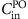and a dc-SQUID (superconducting quantum interference device) termination (Fig. 1a; see Supplementary Fig. 1 and Supplementary Note 1 for the details of the device). The dc-SQUID, working as a controllable inductor, makes the resonant frequency of the resonator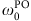dependent on an external magnetic flux through the SQUID loop Φsq (ref. 8). The application of a microwave field at a frequency ωp and a power Pp to the pump line, which is inductively coupled to the SQUID loop, modulates the resonant frequency around its static value. This device has previously been operated as a Jopsehson parametric amplifier9,10. The signal atentering the resonator obtains a parametric gain produced by the pump at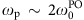and is reflected back along the signal line. This device also works as a parametric oscillator when it is operated at Pp above the threshold Pp0 determined by the photon decay rate of the resonator11,12. Namely, it generates an output microwave field at ωp/2 even without any signal injection. The output can be one of the two degenerate oscillatory states (0π and 1π states) that differ solely by a relative phase shift π.

When an additional signal at ~ωp/2 with a power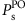, which we call the locking signal (LS), is injected into the parametric oscillator, the degeneracy of the two oscillatory states is lifted13. Such degeneracy lifting has been demonstrated in other physical systems such as magneto-optically trapped cold atoms14 and electromechanical systems15. To understand the dynamics, we consider the Hamiltonian of the PPLO including a signal port for the LS and a fictitious loss port for internal loss of the resonator, namely,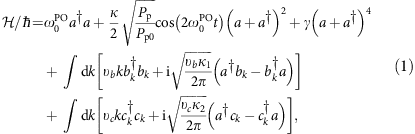where γ represents the nonlinearity of the Josephson junction (JJ), a is the annihilation operator for the resonator, bk (ck) is the annihilation operator for the photon in the signal (loss) port with a wave number k and a velocity υb (υc), κ1 (κ2) represents the coupling strength between the resonator and the signal (loss) port and κ=κ1+κ2. The equations of motion for the classical resonator field in a frame rotating atare obtained from the Hamiltonian by setting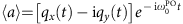and are given by (ref. 16; also see Supplementary Note 2 for derivation)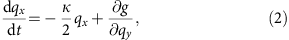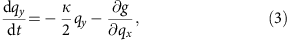where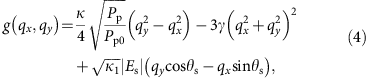and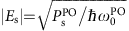and θs are the amplitude and phase of the LS, respectively. In the absence of the first term (oscillator’s friction term) on the right-hand side, equations (2) and (3) are in the form of Hamilton’s equations of motion with the Hamiltonian g(qx, qy), whose minima correspond to 0π and 1π states16. As shown in Fig. 1b, g(qx, 0) is symmetric with respect to qx when there is no LS (|Es|=0), and 0π and 1π states are degenerate. When we apply LS, it gives a tilt to the double well, which is proportional to |Es| sin θs (Fig. 1b,c). This lifts the degeneracy, and the PPLO, initially at qx, qy~0, preferably evolves into one of the two states. This is how the amplitude and the phase of LS control the output state of the PPLO.

### Output of PPLO

Now we show the experimental results. Figure 2a shows the output power of the PPLO operated at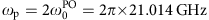(solid blue circles) as a function of Pp in the absence of LS injection. For each Pp, the microwave field is continuously applied to the pump port and the output signal at ωp/2 is measured by a spectrum analyser. Note that power levels stated in this work are referred to the corresponding ports on the chip. The steep increase of the output power at Pp~−65 dBm indicates the onset of the parametric oscillation. This is further confirmed by detecting the response to the pulsed pump. Figure 2b shows the histogram of the demodulated amplitude and phase of the output signal plotted in the quadrature (IQ) plane. For each application of the pump pulse with an amplitude of Pp=−62 dBm, we recorded the output pulse and extracted its amplitude and phase by averaging for 100 ns. The two distribution peaks correspond to 0π and 1π states. They have equal amplitude but different phases shifted by π, and are observed with equal probabilities as expected.

Next we perform a similar measurement, but include LS injection. Figure 2c shows the histogram of the demodulated phase of the output signal as a function of the LS phase. While continuously injecting LS with a phase θs and a power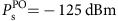, we applied a pulsed pump with the same duration and the amplitude as in Fig. 2b, and extracted the phase of the output signal. As exemplified by the cross-section along the dashed line, the probabilities of obtaining the two states are no longer equal and depend on θs. In Fig. 2d, we plot the probability of 0π state as a function of θs for differents. The probability shows sinusoidal dependence whenis small. As we increase, the modulation amplitude (ΔP0π) also increases and finally reaches unity. We define (1−ΔP0π)/2 as a non-locking error and plot it as a function ofin Fig. 2e using blue circles. Whenis larger than ~−125 dBm (corresponding to NPO of 0.9), the non-locking error becomes negligible. We also simulated the non-locking error by solving a master equation17 based on the Hamiltonian (equation (1)) and plot it by red curve (for details, see Supplementary Note 2 and Supplementary Figs 2 and 3). The only assumption here is the pump threshold Pp0, which is set to be −64.0 dBm. The agreement between the theory and the experiment is fairly good.

### Demodulation of BPSK signal

The above result indicates the PPLO is a phase detector sensitive to very small microwave powers of the order of a femtowatt. To demonstrate this, we generate a signal digitally modulated by BPSK, a scheme commonly used in modern telecommunications18, and demodulate it by using a PPLO. Figure 3a shows the sequence of the experiment. The generated signal has a fixed carrier frequency of 10.503 GHz, fixed power of −125 dBm and a phase that is digitally modulated by π every 500 ns. This means that the signal carries alternating binary bits with a baseband frequency of 2 MHz. Synchronously, we apply pump pulses with the duration of 300 ns and the amplitude of −62 dBm. Figure 3b shows the superposed time traces of the PPLO output. It shows successful demodulation of the input signal except rare errors as exemplified in the figure. We sent a total of 2.4 × 104 bits and detected four errors, corresponding to the error rate of 1.7 × 10−4. As studied in the parametron for its clock speed19, the response time of a PPLO decreases rapidly as we increase Pp and. In the present study, we could make it comparable to the cavity decay time (κ/2)−1 (see Supplementary Fig. 4). This time, together with the integration time to extract the output phase (100 ns in the present study), determines the upper limit for the baseband frequency of the BPSK signal to be demodulated, namely, the bandwidth of the PPLO as a phase detector.

Now we apply this phase discrimination capability to the dispersive readout of a qubit. Figure 4a shows the measurement set-up. A chip containing a 3-JJ superconducting flux qubit capacitively coupled to a CPW resonator (readout resonator) is connected to the PPLO via circulators. The flux qubit is biased at Φq=0.5Φ0, where the qubit transition frequency from |0› to |1› states is 5.510 GHz. Figure 4b shows the phase rotation of the reflection coefficient around the resonant frequency of the readout resonator (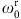) when the qubit is in |0› (blue) and |1› (green). The shift between the two curves is due to the dispersive coupling between the qubit and the readout resonator20. We set ωs to be 10.193 GHz, such that the qubit states are mapped onto two phases of the reflected microwave differing by π.

We discriminate the two states by using the PPLO and the pulse sequence shown in Fig. 4a. We set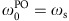, ωp=2ωs (corresponding to open red squares in Fig. 2a) and Pp=−65 dBm. Figure 4c shows the probability of detecting the 0π state as a function of the phase of the readout microwave pulse θs (similar to Fig. 2d) with the qubit control π pulse off (blue) and on (green). The power of the readout microwave field at the input of the readout resonator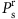is −120 dBm, which corresponds to the mean photon number in the readout resonator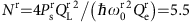. Reflecting the π-phase difference of the reflected microwave field, the two curves are out of phase and their difference corresponds to the fidelity of the qubit readout, which is maximized at −2.0 and 1.2 rad to be 89%.

We further maximized the fidelity by tuning tp and adding a short spike in the beginning of the readout pulse, and measured Rabi oscillations as shown in Fig. 4d, where the length tc and the amplitude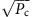of the qubit control pulse are swept. Figure 4e shows the Rabi-oscillation measurement along the dashed line in Fig. 4d. The contrast of the Rabi oscillations is 90.7%. We attribute the sources of the error to incomplete initialization of the qubit by 2.6% and to qubit energy relaxation (including the gate error of the π pulse), which adds 6.7%. Note that the non-zero minimum of the blue curve in Fig. 4c is due to incomplete initialization of the qubit and not the non-locking error. The non-locking error is confirmed to be negligible for both states of the qubit (see Supplementary Note 1 and Supplementary Figs 5 and 6 for the details).

## Discussion

As stated above, Nr of 5.5 is large enough to make the non-locking error of PPLO negligible. It is at the same time small enough for the qubit to avoid readout backaction. By sweeping tp, we can measure T1 of the qubit, while populating photons in the readout resonator. It is measured to be 690 ns (see Supplementary Fig. 7), which agrees with T1 obtained from the independent ensemble-averaged measurement using a standard pulse sequence, namely, a π pulse followed by delayed readout. This indicates the non-destructive character of the present readout scheme.

Another characteristic of the present readout scheme is its latching property. Once the qubit state is mapped to the oscillator state of PPLO, the information can be maintained, regardless of a subsequent qubit state transition, as long as the pump is turned on. We demonstrate this in Fig. 4f, in which the readout fidelity is plotted as a function of td. Even at td=6 μs, at which the qubit has totally decayed, we do not lose readout fidelity.

The present scheme enables fast, latching-type and single-shot readout of the qubit. In this sense, it is similar to schemes using a Josephson bifurcation amplifier6, Josephson-chirped amplifier21 and period-doubling bifurcation22, where the qubit is directly coupled to a nonlinear resonator. However, in the present scheme, the mean photon number in the resonator to which the qubit is coupled can be kept small (of order unity) regardless of the result of the readout. In this sense, it is similar to schemes where a qubit is coupled to a linear resonator, followed by an ultra-low-noise amplifier such as Jopsehson parametric amplifier to achieve single-shot readout10. Thus, the present scheme has combined advantages of both linear and nonlinear resonators and can be useful in quantum error-correction protocols such as the surface code23.